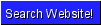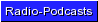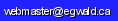Egwald Mathematics: Linear Algebra, Geometry, Optimal Control Theory, Operations Research, Statistics and Econometrics, Nonlinear Dynamics by Elmer G. Wiens Egwald's popular web pages are provided without cost to users. Please show your support by joining Egwald Web Services as a Facebook Fan:Follow Elmer Wiens on Twitter:A. Egwald Mathematics: Linear Algebra Interactive webpages that will help one to understand linear algebra and to solve linear algebra problems. 1. Investigate the different types of polynomials, their graphs, and their roots: polynomials and polynomial roots. 2. Investigate the properties of vectors, vector spaces, and linear transformations on the vectors webpage. 3. Investigate matrices, matrix operations, orthogonal matrices, determinants, matrix inverses, eigenvalues and eigenvectors, generalized eigenvectors and the Jordan Normal Form, along with some important matrix decompositions on the matrices webpage. Matrix decompositions available include Gauss, Gram-Schmidt, Householder, Jacobi (Givens), and singular value decomposition. 4. Solve systems of linear equations, including underdetermined, overdetermined, and square matrix systems. Determine the particular and general solution of systems of linear equations with fewer rows than columns using the Echelon and Singular Value Decomposition algorithms. See the relationship between the image and kernel of a matrix. Use the "normal equations" and singular value decomposition methods to obtain the least squares solution to overdetermined systems of equations. Solve square matrix systems with the Gauss-Jordan, Gaussian, A = QR, and singular value decomposition methods. 5. Solve systems of linear differential equations, using the linear algebra from the preceeding pages. Includes a detailed section on the types of equilibrium for a 2 by 2 system of linear differential equations. B. Egwald Mathematics: Geometry 1. A three dimensional house that you can rotate by adjusting its spherical co-ordinates: 3D-House. 2. The trajectory of a projectile fired from a gun that is mounted on a cliff: Projectile. 3. The natural and reciprocal trigonometric functions - defined and graphed: Trigonometric functions. 4. The inverse functions of the natural and reciprocal trigonometric functions - defined and graphed: Inverse trigonometric functions. 5. The hyperbolic functions - defined and graphed: Hyperbolic functions. 6. Space Curves - the circular helix: Space Curves. C. Egwald Mathematics: Optimal Control 1. Learn how to use the Pontryagin Maximum Principle to solve the rocket car optimal control theory problem. This solution to this optimal control theory problem shows the optimal firing sequence for the rockets mounted at the front and rear of a rocket car that will bring the rocket car to rest at a designated position in the minimum amount of time. I explain and show in diagrams the interaction of the state and adjoint (co-state) variables in the optimal time path of the rocket car. The attainable set and the controllability matrix are also defined and explained. 2. Shoot down a scud missile with a shoulder mounted rocket: Intercept Missile problem. By applying Pontryagin's Maximum Principle to the nonlinear system of equations of motion of the scud missile and shoulder mounted rocket, I derive the optimal control theory minimum time solution to intercepting the scud missile. 3. Solve the famous linearized pendulum (harmonic oscillator) problem using optimal control theory. 4. Solve Linear-Quadratic Optimal Control problems with a finite or infinite time horizon: Linear-Quadratic Regulator. I solve finite time horizon optimal control problems using the Matrix Ricatti Equation, and infinite time optimal control problems using the Algebraic (Steady-State) Ricatti Equation. D. Egwald Mathematics: Operations Research - Linear Programming. 1. Step through a linear programming graphical example. Then solve a problem by hand using the simplex algorithm, or the dual simplex algorithm. 2. Use either the primal simplex tableaux generator, or the dual simplex tableaux generator, to set up and solve your own linear programming problem, with a user-friendly interface. 3. Perform a sensitivity and/or post optimal analysis of the solution to the data of an economics application of linear programming, with a user-friendly interface.             a. The profit maximization decision for a firm.             b. The least cost formula decision for a firm. 4. Step through a linear programming example using the online linear programming solver. 5. Use the online linear programming solver to solve your own L. P. problem. 6. Try your skill on these sample problems. 7. A few linear programming references. 8. Practical use of linear programming: Linear programming is used in the oligopoly / public firm model to derive the bonus rates for the managers of a public firm. See the derivation of the oligopoly/public firm model. E. Egwald Mathematics: Operations Research - Game Theory. 1. Learn the terms and concepts behind game theory in the introduction to game theory. 2. Play a zero sum two person game online. 3. Create and play online your own zero sum two person game. 4. Use the online linear programming solver to solve a zero sum two person game. 5. Learn key concepts from the theory of cooperative, two person non-zero-sum games by stepping through the solution of the "Battle of the Sexes," based on the pioneering work of John Nash. 6. Discover the inescapable dilemma in the Prisoner's Dilemma - a noncooperative, two person non-zero-sum game. 7. What is the free-rider problem? How does it inhibit group rationality? 8. Analyze the Game of Chicken from the James Dean movie, Rebel Without a Cause. 9. The decisions of players in a game where players move sequentially, one after the other, can be modelled with von Neumann and Morgenstern's metagame. The characters in Hermann Melville's short story, Billy Budd, can be viewed as players in a sequence of interdependent, overlapping games, where the events transmitted in the text follow the metagame equilibria of the games. F. Egwald Mathematics: Statistics - Multiple regression analysis.   a. Linear multiple regression. 1. Example: Predicting Canadian Elections using election data for the years 1949-2004: Predicting Canadian Elections. 2. Do your own regression study.   b. Restricted linear regression. 1. Example: Cobb Douglas Production Function 2. Do your own restricted regression study G. Egwald Mathematics: Statistics - Probability and Stochastic Processes.   a. Probability 1. Graphs of the normal and gamma probability distributions. 2. Graphs of the chi-square, Student-t, and F probability distributions. 3. Graphs of the sample mean and variance distributions. 4. Hypotheses testing of means and variances.   b. Stochastic Processes 1. Random Walk. H. Egwald Mathematics: Nonlinear Dynamics One Dimensional Dynamics and Bifurcations One Dimensional Continuous Time Models. Continuous time nonlinear dynamic processes use differential equations to describe the dynamics of the system. 1. Introduction to nonlinear dynamics using the model of population dynamics. 2. The well-known logistics equation of population dynmaics. 3. The general form of a nonlinear differential equation. 4. The local stability of a nonlinear differential equation about a fixed point using linear stability analysis. 5. The major categories of one dimensional continuous time bifurcations: 6. Examples of bifurcation from economic theory: A saddle-node bifurcation in the labour market A transcritical bifurcation in the neoclassical economic growth model A pitchfork bifurcation in the Kaldor business cycle model One Dimensional Discrete Time Models. Discrete time, nonlinear dynamic processes use difference equations to describe the dynamics of the system. 1. Introduction to discrete time, nonlinear dynamic models. 2. The general form of a nonlinear difference equation. 3. The local stability of a nonlinear difference equation about a fixed point using linear stability analysis. 4. The major categories of one dimensional discrete time bifurcations: The one-dimensional, quadratic, logistic map can be used to demonstrate complex, dynamic phenomena that also occur in chaos theory and higher dimensional discrete time systems. 1. Introduction to the logistic map. 2. The linearized stability of the logistic map. 3. The bifurcation analysis of the logistic map. 4. The period doubling bifurcations of the logistic map. 5. The period doubling cascade path to chaos. 6. The logistic map orbit diagram. 7. The third order map f3. 8. Third order map period doubling cascade path to chaos. 9. Click to pop a new window with some interactive logistic map diagrams. 10. Definitions of chaos. The Trygve Haavelmo growth model provides an example of the different dynamical behaviours arising from equivalent models expressed as either differential or difference equations. While the solution trajectories of the continuous time version of the model converge to its fixed point, the solution trajectories of the discrete time version may exhibit chaotic behaviour. 1. In the continuous time version of the model, solution trajectories of the differential equation governing the growth of employment converge to a stable fixed point. 2. The phase diagrams show the solution trajectories for various values of the parameter α of the model's differential equation. 3. In the discrete time version of the model, solution trajectories may follow periodic cycles and eventual exhibit chaotic behaviour as the parameter α of the model's difference equation increase. 4. The phase diagrams show the solution trajectories for various values of the parameter α of the model's difference equation. 5. 5. The bifurcation diagram shows the period doubling cascade path to chaos. Two Dimensional Flows and Phase Diagrams Introduction to two dimensional flows. 1. Dynamics of linear systems of differential equations. 2. Classification of Fixed Points for Linear Systems: stability diagram. 3. The general form of a two dimensional nonlinear system of equations. 4. Determining the fixed points of a two dimensional nonlinear system. 5. The linearized stability of a two dimensional nonlinear system. 6. The Jacobian of a two dimensional nonlinear system. 7. Classification of Fixed Points for Linear Systems: topological diagram. 8. Example of a nonlinear dynamic system: Strogatz example. 9. Example of a nonlinear dynamic system: Boyce and DiPrima. 10. Example of a nonlinear dynamic system: oscillating pendulum. 11. The dynamics diagram of the oscillating pendulum. 12. The Lotka � Volterra � Goodwin Predator-Prey Model predator prey model. Limit Cycles and Two Dimensional Flows Introduction to limit cycles. 1. Example of a nonlinear system with a limit cycle: example. 2. Transformation to polar coordinates. 3. The van der Pol Oscillator as the prototype of a nonlinear system exhibiting limit cycles. 4. Criteria to determine the absence or presence of limit cycles. 5. The Poincaré-Bendixson Theorem. 6. The Kaldor Business Cycle Model. Bifurcations and Two Dimensional Flows Introduction to bifurcations. 1. Prototype bifurcations:           a. saddle-node bifurcation.           b. transcritical bifurcation.           c. pitchfork bifurcation. 2. In Hopf bifurcations, a stable limit cycle emerges — growing from a fixed point (supercritical Hopf bifurcation), or an unstable limit cycle disappears — diminishing towards a fixed point. 3. Two parameter bifurcations and the monkey saddle bifurcation as an example. 4. Global bifurcations:           a. global saddle-node bifurcation.           b. infinite period bifurcation.           c. homoclinic bifurcation. Mathematical Appendix Introduction to mathematical appendix. 1. The direction field and phase portrait provide qualitative information about the solutions to a system of differential equations. 2. The fixed points (equilibrium points) of the nonlinear differential equation system. 3. Fixed points occur at the intersection(s) of the system's nullclines. 4. Nonlinear dynamical systems can have multiple fixed points which may be stable or unstable. 5. The Taylor series expansion of the equations of motion linearizes the system about its fixed points. 6. The Jacobian matrix reveals the dynamics of the nonlinear system. 7. Saddle points may have homoclinic or heteroclinic orbits (solution trajectories). 8. A differential equation system with a first integral is called a conservative system. 9. The Lotka—Volterra predator-prey model as an example of a conservative system. 10. Use index theory to detect the presence and characteristics of fixed points.Copyright © Elmer G. Wiens:   Egwald Web ServicesAll Rights Reserved.    Inquiries